# Functions in AP Calculus: Help and Review Chapter Exam

Exam Instructions:

Choose your answers to the questions and click 'Next' to see the next set of questions. You can skip questions if you would like and come back to them later with the yellow "Go To First Skipped Question" button. When you have completed the practice exam, a green submit button will appear. Click it to see your results. Good luck!

### Page 1

#### Question 3 3. What is the domain of the function below?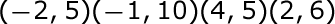### Page 2

#### Question 6 6. What is the inverse function of f(x) = 3(x - 2)3?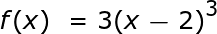#### Question 7 7. Using the graph, find f(f(-2)).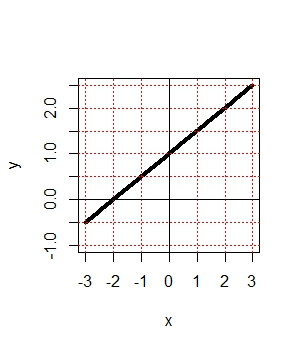#### Question 8 8. If the graph of f(x) is shown below, which choice represents the graph of f(x + 1) + 2?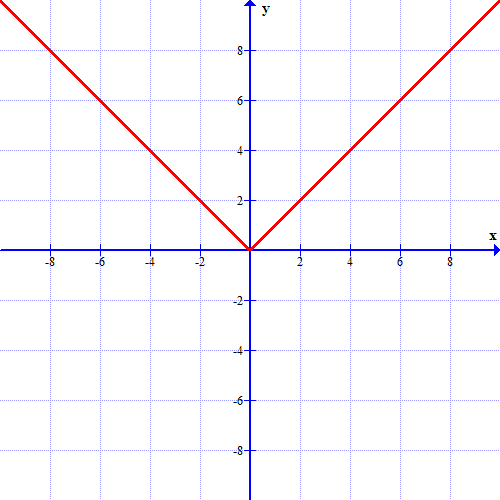### Page 3

#### Question 11 11. Remembering function notation, we want x to be w. Therefore, if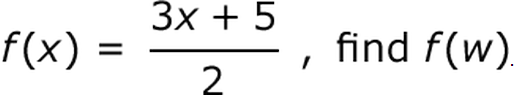#### Question 13 13. In the figure shown below, the graph of the yellow function is a translation of the graph of the blue function. If the expression for the blue function is y(x) = b(x), which of the following is the equation of the yellow function?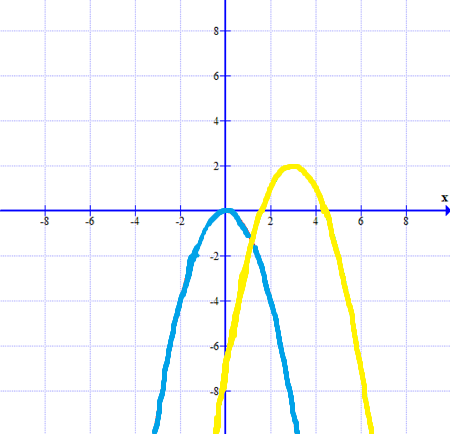#### Question 14 14. What is the range of the function represented by the graph below?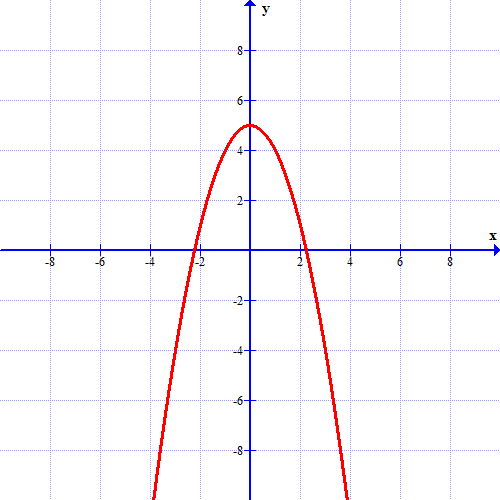### Page 4

#### Question 16 16. Evaluate f(f(x)). [Yes, it says f(f(x)]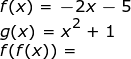#### Question 19 19. Which kind of transformation would change the purple function below into the yellow one?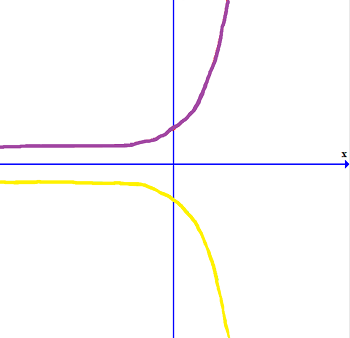### Page 5

#### Question 23 23. The revenue of a company is represented by R(x) and their costs are represented by C(x). What is the profit function R(x) - C(x)?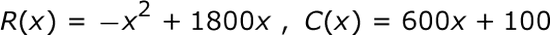### Page 6

#### Question 27 27. Using the function below, evaluate g(7).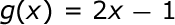#### Functions in AP Calculus: Help and Review Chapter Exam Instructions

Choose your answers to the questions and click 'Next' to see the next set of questions. You can skip questions if you would like and come back to them later with the yellow "Go To First Skipped Question" button. When you have completed the practice exam, a green submit button will appear. Click it to see your results. Good luck!

Support